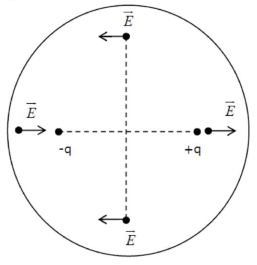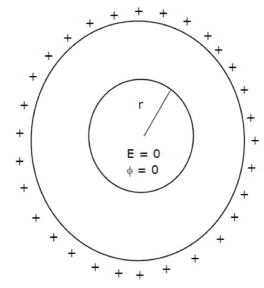# Given below are two statements :Question:

Given below are two statements :

Statement - I : An electric dipole is placed at the centre of a hollow sphere. The flux of electric field through the sphere is zero but the electric field is not zero anywhere in the sphere.

Statement - II : If $\mathrm{R}$ is the radius of a solid metallic sphere and $\mathrm{Q}$ be the total charge on it.

The electric field at any point on the spherical surface of radius $r( flux passing through this closed spherical surface of radius$r$is not zero. In the light of the above statements. Choose the correct answerfrom the option given below : Option : 1. (1) Statement I is true but Statement II is false 2. (2) Statement I is false but Statement II is true 3. (3) Both Statement I and Statement II are true 4. (4) Both Statement I and Statement II are false Correct Option: 1 Solution: (1)Statement$-1 \rightarrow$CorrectStatement$-2 \rightarrow\$ Incorrect